3D Geometry Primer: Chapter 2 - Issue 02 - More About Lines by (03 September 2002) Return to The Archives
 IntroductionToday we have a massive issue. It's bigger than ever, and contains more math than ever. Very scary sometimes, since we'll prove things! If you're scared, if you're afraid of terrible beasts under your bed, if you just can't handle the truth, then you may skip the proofs. But if you're brave and willing to go where no one has gone before (I'm not one), then you should try to understand them. It will widen your vision on certain formulas that you already may know, but never realized why they are what they are. Once you understand that, you can go even beyond that and find out things no one ever told you. And that's the beauty of math. Anything can happen, because it's all in your mind!"It has been shown as proof positive that carefully prepared chocolate is as healthful as a food as it is pleasant; that it is nourishing and easily digested ... that it is above all helpful to people who must do a great deal of mental work.", Anthelme Brillat-Savarin (1755–1826), French magistrate and gastronomeLast week, we found out that a good parametric equation of a line consists of a support point S and a (normalized) direction vector D. All points P of the line were then given by P(t) = S + t*D , t Î ]–Ą, +Ą[. Today, we're going to measure distances between points/lines.

 (I) Distance Between Two Points On The Same LineIf you want to know the distance between 2 points on a single line d, you can of course use Pythagoras. But there's also another way that might be much cheaper.Consider two points P1 and P2 on a line d with parametric equation P = S + t*D. Let us assume that we already know the values t1 and t2 that generate our points P1 and P2 respectively: i.e. we know that P1 = S + t1*D and P2 = S + t2*D.To calculate the distance between P1 and P2, we try the same thing as we did with Pythagoras. But now, if we replace P1 and P2 by their parametric representation, we will get: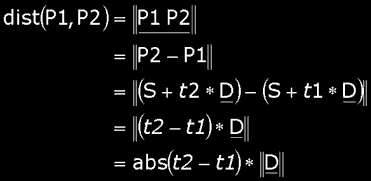And what's more: if D is normalized then we have: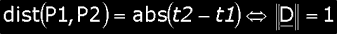So, to determine the distance between two points on the line, you only have to subtract their parameters (of course, you have to take the absolute value of it, and this only counts if the D is normalized). Notice that this is only useful if you already know the parameters t1 and t2. Otherwise, you first need to calculate them, so its more favorable to use Pythagoras in that case.

 (II) Possible Relationshipsa) Point – LineAgain very simple: A point lies on the line, or it does not.b) Line – LineWe have four possibilities: coincident, parallel, crossing and intersecting lines.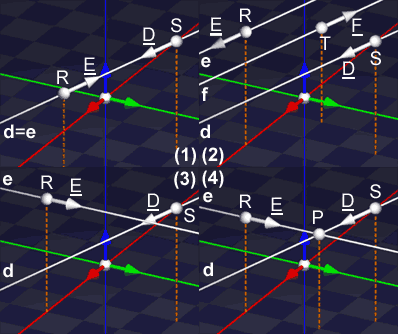Coincident Lines: Some lines have all their points in common. And basicly they are the same line. Each point of one line is also a point of the other one, and vice-versa. Although it might not seem to at first: d has its support point P and direction vector D while e uses R and E, and yet d and e are the same line: they are coincident.Conditions to be coincident: (1) Direction vectors must have same direction (they can have a different sense however). (2) At least one point of one line (e.g. the support point) must be on the second line. (alternative condition for (2): the distance between both lines is zero)Parallel Lines: Some lines do have parallel direction vectors, but don't have any points in common. They are called parallel lines. Notice that coincident lines can be seen as a special case of parallel lines: coincident lines are parallel lines that share all points.Conditions to be parallel: (1) Direction vectors must have same direction. (2) At least one point of one line is NOT on the second line. (alternative test for (2): the distance between both lines is NOT zero)Notice that if the direction vectors are parallel, the lines share or all or no points.Crossing Lines: Most lines don't have any points in common and don't have parallel directions. The one goes over or under the other without touching. This is called crossing. Crossing lines share zero points.Conditions to be crossing: (1) Direction vectors can not have the same direction. (2) The lines don't have any points in common: the distance between both lines is bigger than zero.Intersecting Lines: Some lines have only one point in common. We say that they intersect. They have exactly 1 point in common: the intersection point.Conditions to be intersecting: (1) Direction vectors can not have the same direction. (2) The lines have exactly 1 point in common: the distance between both lines is zero.

 (III) Distance From An Arbitrary Point To A Linea) The FormulaThe distance from a point P to a line d <–> Q = S + t * D, is per definition the distance to that point of d that is closest to P. It is the length of the shortest path you have to travel to get from P to the line. If you know that point, then it's easy: the distance from P to d is the distance from P to that point. But how do you get that closest point?It seems that you need to project P orthogonally onto d. This means that the line through P and P' (we'll call that closest point P', because it's the image of P onto d). How you do that, is explained in chapter I, issue 3, paragraph (i) a) perpendicular projection (it should have been called orthogonal projection, but hey :)Anyway, it goes like this: you project the vector SP onto the direction of D to get SP' (don't forget that ||D|| = 1):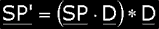Then you add this to S and you get P' = S + SP', the point we're after. Now the only thing you still need to do is to take the vector PP', calculate its norm and you know the distance: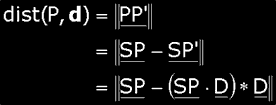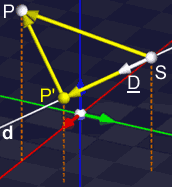b) Mathematical ProofHere I'm going to prove that the formula I just gave you is indeed the correct one, that it gives you the distance to the closest point of the line. Normally, this would have been very mathematical, but fortunately for you, Nick slapped me with a very old pain français (it still hurts) and showed me a much easier way. Thank you Nick ...OK, what was this magic thing by Nick? Right triangles! You all know that, don't you? Triangles with exactly one angle of 90 degrees. We know of right triangles that the hypotenuse is always the longest edge, longer than both edges that share the right angle. Now, we'll use that to prove our formula.If we look very close to that image, we'll see that the points P, P' and S form a right triangle with P' the right angle. This is, of course, because P' is the orthogonal projection of P onto d, and that demands a right angle in P'. This way, we already know that the distance between P and P' is shorter than between P and S: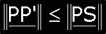But what's more: you can replace S by *any* point Q(t) of d. And still P, P' and Q will be a right triangle with P' the right angle. This means that for *every* point Q(t) of d, the distance from P to P' will be shorter than from P to Q(t).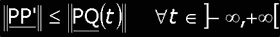Hence, P' is the point of d that is closest to P. Tadaa! This proves that ||PP'|| indeed is the distance from the point P to the line d. The formula is correct.ExerciseThe line d has starting point S(2, 3, 3) and direction vector D(2/3, –2/3, 1/3). The point from where we want to know the distance is P(3, 0, 4). See for yourself if you can find the distance between P and d. The answer should be 1.41.

 (IV) Distance Between Two LinesIf you have two lines d and e (with support points S and R, and direction vectors D and E respectively), then the distance dist(d, e) between those lines is the distance between the closest 2 points. In the image, I've called those points P and Q. There are no other points of d and e that are closer together. Hence the shortest distance between lines d and e is the one between P and Q. Once we know these points, we simply need to calculate the distance between them, and you know dist(d, e).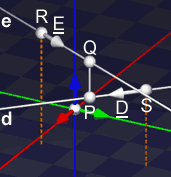a) The Formula:Fortunately, we don't really need to know these points. But unfortunately, there's a special case! The general formula can't be use on parallel lines, because it's based on a cross product of the direction vectors of the lines. And if these direction vectors are parallel, our cross product will be 0 (zero). That would mean that the formula would return that the distance between two prallel lines is always 0 (zero). Nonsens of course! Fortunately, there's a work around. Anyway, here you get the formula and a piece of pseudocode:```float dist(line d <-> S + t * D, line e <-> R + u * E) { SR = R - S; N = D × E; if (N == 0) { // special case: direction vectors are parallel // we have parallel or coincident lines here return ||SR – (SR · D) * D||; } else { // normal case: direction vectors aren't parallel // we have crossing or intersecting lines here return abs(SR · N); } }```b) Proof:If you don't want to know why this formula is what it is, or you're too scared of knowing the truth, you can skip the next part and go directly to the next paragraph. Here I'm going to prove both parts of the formula:The Non-Parallel Part:Consider the following 3 vectors D, E and D × E. As long as D and E are not parallel, D × E will be perpendicular to both D and E. We assume that D and E are not parallel, hence these vectors are linear independent and we can construct a new vector base for our space: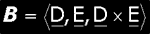And now we can write each vector V in space as a unique linear combination of these 3 new base vectors. For each vector V you'll find a unique trio a, b and c. There's no other trio that results in V: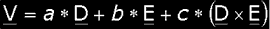You can do this also for vectors between point P(u) of line d and point Q(v) of line E. These vectors PQ are function of both u and v, and so do a, b and c: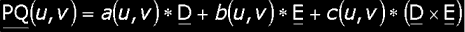Now, the beauty is that c is in reality not a function of u and v. It's a constant for all points P and Q. Can this be proven? Certainly. We know that P, Q and PQ are functions of u and v: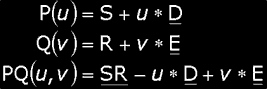We can write RS as a linear combination of D, E and D × E. In this combination, we'll find three values that are independent on u and v. This is true because nor R nor S depends on them. We use that combination to rewrite PQ(u, v):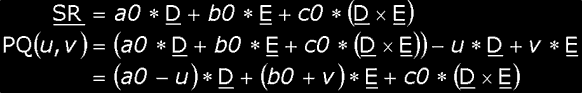Note: In an orthonormal base you would be able to find a0, b0 and c0 by projecting RS orthogonal onto the base vectors. However, this cannot be done here, because D is not necessarely orthogonal to E, we have a non-orthogonal base! However, lucky for us, the only one we'll really need to know is c0, and for that one it can be done! This is because D × E is orthogonal to D and E. And since D × E is a unit vector this results in: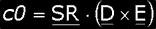How does this help us? Just as for the distance between a point and a line, we'll construct a function f(u, v) that tells us the distance between the 2 points P(u) and Q(v). And then, we'll use a little house-, garden- and kitchenreasoning to find the minimum of that function. And that will be the distance, we're looking for ...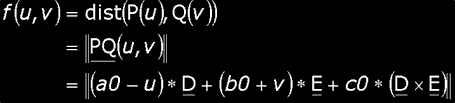Consider the situation for which u = a0 and v = –b0: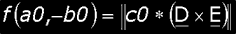Now I tell you that this is the minimum of the function. Can I prove it? Imagine that u ą a0 but still v = –b0. We get: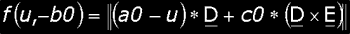You can see the three vectors (a0 – u) * D, c0 * (D × E) and (a0 – u) * D + c0 * (D × E) as a triangle. And since D is orthogonal to D × E we have a right-angled triange with (a0 – u) * D + c0 * (D × E) as hypotenuse (the edge opposite the right angle).In a right-angled triangle, the hypotenuse is always the longest edge, and here it has length f(u, –b0). One of the other two edges is c0 * (D × E). This means, that from the moment u is not a0, we know that the distance between our points P(u) and Q(v) is longer than our proposed minimum || c0 * (D × E) ||.Since D and E are mostly normalized, we know that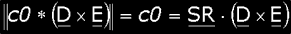This is the distance between two non-parallel lines.The Parallel Part:As I've already said, we can't use previous method to calculate the distance between two parallel lines, because D × E would result in the null vector. But as always, there's a solution to this problem.d and e are parallel. It means they have parallel direction vectors and that we can write E = c * D. That helps us to rewrite the equations of the lines a little: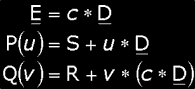we now can use a new parameter v' for the second line: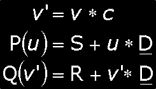Now we'll calculate the distance form any point Q(v') on e to e. We'll do that with the previous derived function. Then we substitute the real meaning of Q(v') and simplify: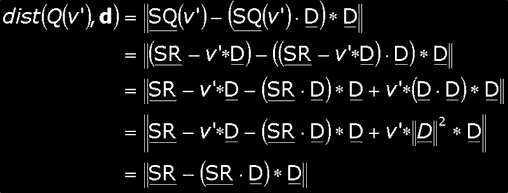We can see that this is constant for all v' and that it is equal of the distance from point R to line d. And for each point Q(v') of e, this is the minimum distance from that point to the line d. This means that this is also the minimum distance between both lines d and e.

 (V) Intersection Of Two LinesThe intersection of two lines (or two objects in general) is always a bit tricky, because you can't simply return one point as the intersection. For example: two lines can have no points in common (crossing lines, parallel lines), all their points in common (coincident lines) or exactly one point in common (intersecting lines)The first situation is easy to detect: if the distance between the two lines is not zero, then there's a gap between both lines. And that means they can't have any point in common. And the answer is as simple: there are no intersection points.The second situation is almost as easy to detect: if the distance between the lines is zero *but* the direction vectors are parallel, we have coincident lines. In that case the answer is also simple: all the points of D (or E) are intersection points, since they have all points in common.If it isn't the first or second situation, we are sure we're in the last situation: intersection lines. And only then we can return what we're looking for: the intersection point.This is a lot of information to return through the return-value of a function. I've decided to return the number of intersection points through the return-value. And if there is an intersection point, I return the parameters for that point through t and u. These parameters will give you the exact intersection point if you substitute one in its equation (of course, both equations will result in the same point, that's the definition of the intersection point :). Why don't I immediately return the intersection point instead of t and u? This is because many times you need the parameter instead of the exact point, and it's easier to find the exact point using the parameter than finding the parameter using the intersection point.First, I'll give you the pseudo-code. And after that, I'll explain how you find t and u.``` // --------------------------------------------------------------------- // FUNCTION TO FIND THE INTERSECTION OF TWO LINES. // // Several cases to handle: // crossing or parallel lines: return 0, t and u contain no information // coincident lines : return -1, t and u contain no information // intersection lines : return 1, t and u contain parameters of // intersection point for both lines // ---------------------------------------------------------------------int intersection(const line d <-> S + t * D, const line e <-> R + u * E, float& t, float& u) { // distance between d and e must be zero to have points in common if (dist(d, e) > 0) return 0; // cross product of direction vectors N = D × E; // if direction vectors are parallel, we have coincident lines if (N == 0) return -1; // else, we have exactly one point in common // find the two parameters t and u for it. // vector between origins of lines: SR = R – S; // Solve with cramer // Use minor with largest determinant. if (abs(n3) > abs(n1) && abs(n3) > abs(n2)) { t = (sr1 * e2 - rs2 * e1) / n3; u = – (sr1 * d2 - rs2 * d1) / n3; } else if (abs(n1) > abs(n2)) { t = (sr2 * e3 - rs3 * e2) / n1; u = – (sr2 * d3 - rs3 * d2) / n1; } else { t = (sr3 * e1 - rs1 * e3) / n2; u = – (sr3 * d1 - rs1 * d3) / n2; } return 1; }```Once we know we're dealing with intersecting lines, we still need to know t and u. This is done by demanding that if the correct values are substituted, both equations for d and e result in the same point: the intersection point. Doing this is very easy: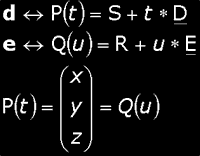or after splitting up each equation of a line in three different equations, one for each component value: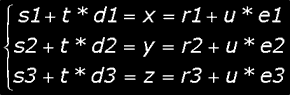This system contains 3 equations and 2 unknowns t and u. Since we already know we'll find exactly one solution, these equations can't be contradictory and that we can leave one equation. For now we'll leave the last one. After some rearranging we become the following: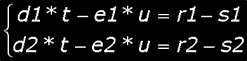We can solve this to t and u by using Cramer and we get the following results (remarks):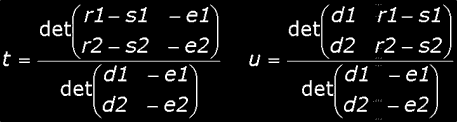Notice that the denominator is nothing else than –n3, the negative of the third component value of N = D × E. If we take this in account and we write SR(sr1, sr2, sr3) = R(r1, r2, r3) – S(s1, s2, s3), then this simplifies to the following: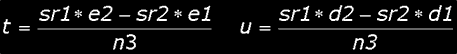In this case, I've chosen to dump the third equation and to continue with the first two. This is not always a good choice. In fact, always dumping the third one can lead to an unsolvable situation in certain cases. When does this happen? Look to the previous solutions for t and u. What happens if n3 becomes 0 (zero)? Precisely. We get a nice division by zero. And we don't want that, do we?To examine this, we'll take a look at the three different outcomes as we leave out each time another equation. The first one leaves out the first equation, the last one the last: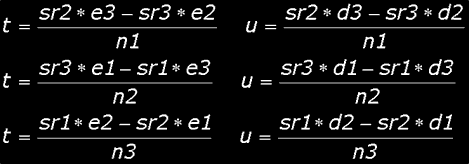All three lead to the same result, in theory. In practice, some may become undefined because their denominator becomes zero. Which one to pick then? I say: choose the one with the largest denominator (in absolute value). If abs(n1) ł abs(n2) and abs(n1) ł abs(n3), then pick the first one. We can be sure that n1 won't be zero, because otherwise we would have the nullvector for N. And since we assumed that D and E are not parallel, we know N can't be the nullvector.Of course, you can make this algorithm a little faster by inlining and hardcoding dist(d, e). In that way, you'll be able to avoid a cross product: you also need N = D × E to calculate the distance between d and eThat's it for our lines. Thank you Nick for proof-reading this.See you all next week, same time, same channel, Bramzistor Radio

 Remarks